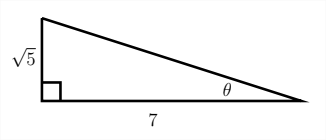# 8.2: The Trigonometric Ratios

$$\newcommand{\vecs}{\overset { \rightharpoonup} {\mathbf{#1}} }$$ $$\newcommand{\vecd}{\overset{-\!-\!\rightharpoonup}{\vphantom{a}\smash {#1}}}$$$$\newcommand{\id}{\mathrm{id}}$$ $$\newcommand{\Span}{\mathrm{span}}$$ $$\newcommand{\kernel}{\mathrm{null}\,}$$ $$\newcommand{\range}{\mathrm{range}\,}$$ $$\newcommand{\RealPart}{\mathrm{Re}}$$ $$\newcommand{\ImaginaryPart}{\mathrm{Im}}$$ $$\newcommand{\Argument}{\mathrm{Arg}}$$ $$\newcommand{\norm}{\| #1 \|}$$ $$\newcommand{\inner}{\langle #1, #2 \rangle}$$ $$\newcommand{\Span}{\mathrm{span}}$$ $$\newcommand{\id}{\mathrm{id}}$$ $$\newcommand{\Span}{\mathrm{span}}$$ $$\newcommand{\kernel}{\mathrm{null}\,}$$ $$\newcommand{\range}{\mathrm{range}\,}$$ $$\newcommand{\RealPart}{\mathrm{Re}}$$ $$\newcommand{\ImaginaryPart}{\mathrm{Im}}$$ $$\newcommand{\Argument}{\mathrm{Arg}}$$ $$\newcommand{\norm}{\| #1 \|}$$ $$\newcommand{\inner}{\langle #1, #2 \rangle}$$ $$\newcommand{\Span}{\mathrm{span}}$$$$\newcommand{\AA}{\unicode[.8,0]{x212B}}$$

There are six common trigonometric ratios that relate the sides of a right triangle to the angles within the triangle. The three standard ratios are the sine, cosine and tangent. These are often abbreviated sin, cos and tan. The other three (cosecant, secant and cotangent) are the reciprocals of the sine, cosine and tangent and are often abbreviated csc, sec, and cot.Given an angle situated in a right triangle, the sine function is defined as the ratio of the side opposite the angle to the hypotenuse, the cosine is defined as the ratio of the side adjacent to the angle to the hypotenuse and the tangent is defined as the ratio of the side opposite the angle to the side adjacent to the angle.
\begin{aligned} \sin \theta &=\frac{o p p}{h y p} \\ \cos \theta &=\frac{a d j}{h y p} \\ \tan \theta &=\frac{o p p}{a d j} \end{aligned} A common mneumonic device to help remember these relationships is
-SOHCAHTOA-which identifies the sin as Opp over Hyp Cos as Adj over Hyp and the Tan as Opp over Adj.

An acute angle placed in the other position of a right triangle would have different oppposite and adjacent sides although the hypotenuse would remain the same.Examples: Trigonometric Ratios
Find $$\sin \theta, \cos \theta$$ and $$\tan \theta$$ for the given angle $$\theta$$In order to find the sin and cos of the angle $$\theta$$, we must first find the hypotenuse by using the Pythagorean Theorem $$\left(a^{2}+b^{2}=c^{2}\right)$$

since we know the legs of the triangle, we can substitute these values for $$a$$ and $$b$$ in the Pythagorean Theorem:
$\begin{array}{c} 3^{2}+5^{2}=c^{2} \\ 9+25=c^{2} \\ 34=c^{2} \\ \sqrt{34}=c \end{array}$ Now that we know the hypotenuse $$(\sqrt{34}),$$ we can determine the sin, cos and tan for the angle $$\theta$$
\begin{aligned} \sin \theta &=\frac{3}{\sqrt{34}} \\ \cos \theta &=\frac{5}{\sqrt{34}} \\ \tan \theta &=\frac{3}{5} \end{aligned} Find $$\sin \theta, \cos \theta$$ and $$\tan \theta$$ for the given angle $$\theta$$Again, in order to find the sin, cos and tan of the angle $$\theta,$$ we must find the missing side of the triangle by using the Pythagorean Theorem. since, in this case, we know the hypotenuse and one of the legs, the value of the hypotenuse must be substituted for $$c$$ and the length of the leg we're given can be substituted for either
$$a$$ or $$b$$

$\begin{array}{c} 4^{2}+b^{2}=9^{2} \\ 16+b^{2}=81 \\ b^{2}=65 \\ b=\sqrt{65} \end{array}$ Now that we know the length of the other leg of the triangle $$(\sqrt{65}),$$ we can determine the sin, cos and tan for the angle $$\theta$$
\begin{aligned} \sin \theta &=\frac{\sqrt{65}}{9} \\ \cos \theta &=\frac{4}{9} \\ \tan \theta &=\frac{\sqrt{65}}{4} \end{aligned} In addition to the examples above, if we are given the value of one of the trigonometric ratios, we can find the value of the other two.
Example
Given that $$\cos \theta=\frac{1}{3},$$ find $$\sin \theta$$ and $$\tan \theta$$
Given the information about the cosine of the angle $$\theta,$$ we can create a triangle that will allow us to find $$\sin \theta$$ and $$\tan \theta$$Using the Pythagorean Theorem, we can find the missing side of the triangle:
$\begin{array}{c} a^{2}+1^{2}=3^{2} \\ a^{2}+1=9 \end{array}$ $$a^{2}=8$$
$$a=\sqrt{8}=2 \sqrt{2}$$
Then $$\sin \theta=\frac{\sqrt{8}}{3}$$ and $$\tan \theta=\frac{\sqrt{8}}{1}=\sqrt{8}$$
You might say to yourself, "Wait a minute, just because the cosine of the angle $$\theta$$ is $$\frac{1}{3},$$ that doesn't necessarily mean that the sides of the triangle are 1 and $$3,$$ they could be 2 and $$6,$$ or 3 and 9 or any values $$n$$ and $$3 n . "$$

This is true, and if the sides are expressed as $$n$$ and $$3 n,$$ then the missing side would be $$n \sqrt{8},$$ so that whenever we find a trigonometric ratio, the $$n^{\prime}$$ s will cancel out, so we just leave them out to begin with and call the sides 1 and 3
Example
Given that $$\tan \theta=\frac{\sqrt{5}}{7},$$ find $$\sin \theta$$ and $$\cos \theta$$
First we'll take the infomation about the tangent and use this to draw a triangle.Then use the Pythagorean Theorem to find the missing side of the triangle:
$\begin{array}{c} \sqrt{5}^{2}+7^{2}=c^{2} \\ 5+49=c^{2} \\ 54=c^{2} \\ \sqrt{54}=3 \sqrt{6}=c \end{array}$ So then:
$\sin \theta=\frac{\sqrt{5}}{\sqrt{54}}=\sqrt{\frac{5}{54}}$ $\cos \theta=\frac{7}{\sqrt{54}}=\frac{7}{3 \sqrt{6}}$

Exercises 1.2
Find $$\sin \theta, \cos \theta$$ and $$\tan \theta$$ for the given triangles.Use the information given to find the other two trigonometric ratios.
11. $$\quad \tan \theta=\frac{1}{2}$$
12. $$\quad \sin \theta=\frac{3}{4}$$
13. $$\quad \cos \theta=\frac{3}{\sqrt{20}}$$
14. $$\quad \tan \theta=2$$
15. $$\sin \theta=\frac{5}{\sqrt{40}}$$
16. $$\sin \theta=\frac{7}{10}$$
17. $$\cos \theta=\frac{9}{40}$$
18. $$\quad \tan \theta=\sqrt{3}$$
19. $$\cos \theta=\frac{1}{2}$$
20. $$\cos \theta=\frac{3}{7}$$
21. $$\sin \theta=\frac{\sqrt{5}}{7}$$
22. $$\quad \tan \theta=1.5$$

This page titled 8.2: The Trigonometric Ratios is shared under a CC BY-NC-SA 4.0 license and was authored, remixed, and/or curated by Richard W. Beveridge.